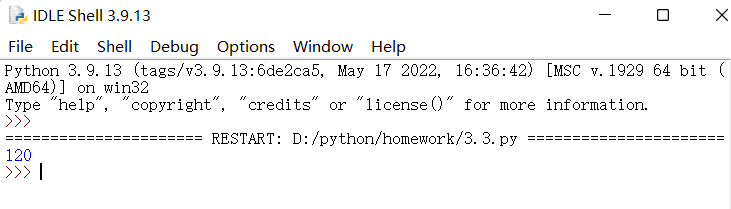# 作业要求：

1. 在自己的Python编程环境里，编程实现上述三道练习题，最好和参考答案代码不一样。

2. 测试运行你自己编写的代码和参考答案代码。

3. 如果测试运行时候，你的代码出现Bug，分析原因并调试代码。

## 一 、函数定义及使用

### 样例

``````def arithmetic_operations(x, y):
if y == 0:
return None
sum = x + y
difference = x - y
product = x * y
quotient = round(x / y, 2)
return sum, difference, product, quotient
result = arithmetic_operations(5, 3)
print(result)
``````

## 二、Lambda函数

### 样例

``````greater_than_n = lambda lst, n: [x for x in lst if x > n]
lst = [1,3,5,7,9,11]
n = 5
result = greater_than_n(lst, n)
print(result)``````

## 三、递归函数

### 样例

``````def factorial(n):
result = 1
for i in range(1, n+1):
result *= i
return result
n = 5
result = factorial(n)
print(result)``````...全文
43 回复 打赏 收藏 转发到动态 举报Python程序设计作业.pdf
Python程序设计作业.pdf2023（春）Python程序设计作业3： 函数应用
if y == 0:else:x, y = 4，6二、Lambda函数n = 7三、递归函数if n == 1:return 1else:n = 5。2020-11-08 Windows应用程序设计作业Python版实现）（一）简单语法
Windows应用程序设计作业Python版实现） 作业1-语法 1、任给一个自然数，若为偶数除以2，若为奇数则乘3加1，得到一个新的自然数后按照上面的法则继续演算，若干次后得到的结果必然为1。请编程验证。 ''' 函数名称：test_for_rule 函数功能：任给一个自然数，若为偶数除以2，若为奇数则乘3加1， 得到一个新的自然数后按照上面的法则继续演算，若干次后得到的结果必然为1。 最新版本：2020/10/20 ''' def test_for_rule(n, change_li2023（春）Python程序设计作业5：Pandas基础技能及综合应用
【代码】2023（春）Python程序设计作业5：Pandas基础技能及综合应用Python程序设计（苏州大学）_第五章单元测试及作业_答案_中国大学MOOC
Python程序设计（苏州大学） 第五章单元测试及作业_答案 中国大学MOOC 目录Python程序设计（苏州大学）第五章单元测试及作业_答案中国大学MOOC单元测试作业 单元测试 1单选(4分)关于函数，以下选项中描述错误的是 A.函数是一段具有特定功能的、可重用的语句组 B.使用函数的主要目的是降低编程难度和代码重用 C.Python使用del保留字定义一个函数 D.函数能完成特定的功能，对函数的使用不需要了解函数内部实现原理，只要了解函数的输入输出方式即可 2单选(4分)‌关于eval函数，以下选项中

162发帖与我相关我的任务
python 高校 江苏省·南通市• 近7日
• 近30日
• 至今